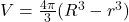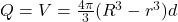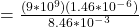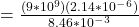## Calculate the magnitude of the electric field inside the solid at a distance of 9.20 cmcm from the center of the cavity. Express your answer

Question

Calculate the magnitude of the electric field inside the solid at a distance of 9.20 cmcm from the center of the cavity. Express your answer with the appropriate units.

in progress 0
5 months 2021-09-05T09:18:45+00:00 1 Answers 26 views 0

A point charge of -2.14uC  is located in the center of a spherical cavity of radius 6.55cm  inside an insulating spherical charged solid. The charge density in the solid is 7.35×10−4 C/m^3.

a) Calculate the magnitude of the electric field inside the solid at a distance of 9.20cm  from the center of the cavity.

==7.3  × 10⁻⁵N/C

Explanation:

A point charge ,q = -2.14uC = – 2.14 × 10⁻⁶

cavity of radius , r = 6.55cm = 6.55 × 10⁻²m

charge density in the solid = 7.35×10⁻⁴ C/m³

Distance from the center of the cavity,R = 9.20cm = 9.2 × 10⁻²m

Volume of shell of charge =Charge on the shell ,Q = V × dQ = 4.1888 × ((9.2 × 10⁻²)³ – (6.55 × 10⁻²)³) × 7.35 × 10⁻⁴

Q = 1.47 × 10⁻⁶N/C

Electric field at 9.2× 10⁻²m due to shell

E1 = kQ/R²= 1.563 × 10⁻⁶N/C

Electric field at 9.2 × 10⁻²m due to q at center

E2= 2.276 × 10⁻⁶N/C

magnitude of the electric field inside the solid at a distance of 9.50cm from the center of the cavity

= E2- E1

= (2.276 × 10⁻⁶) – (1.563 × 10⁻⁶)N/C

= 0.73 × 10⁻⁶N/C

= 7.3  × 10⁻⁵N/C